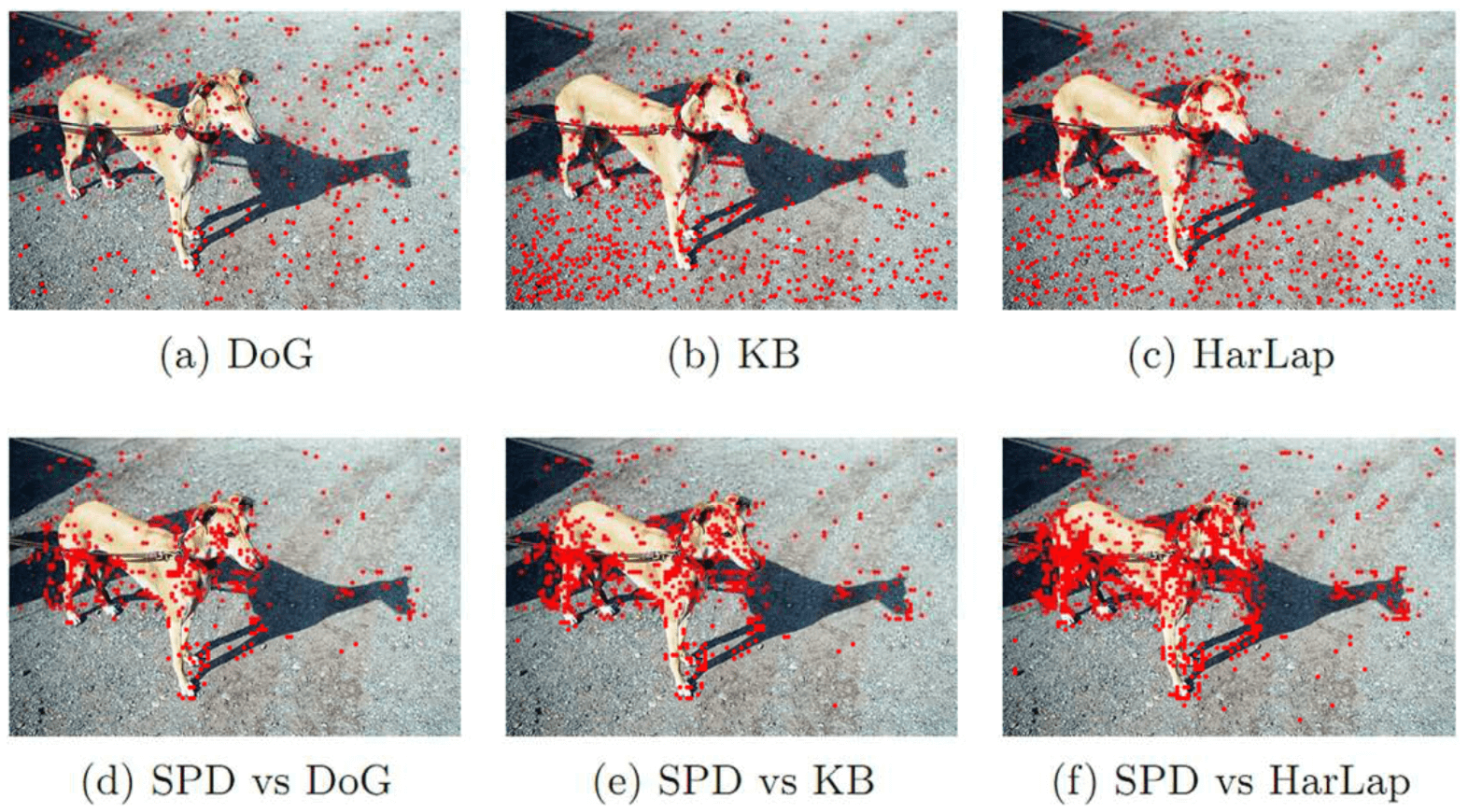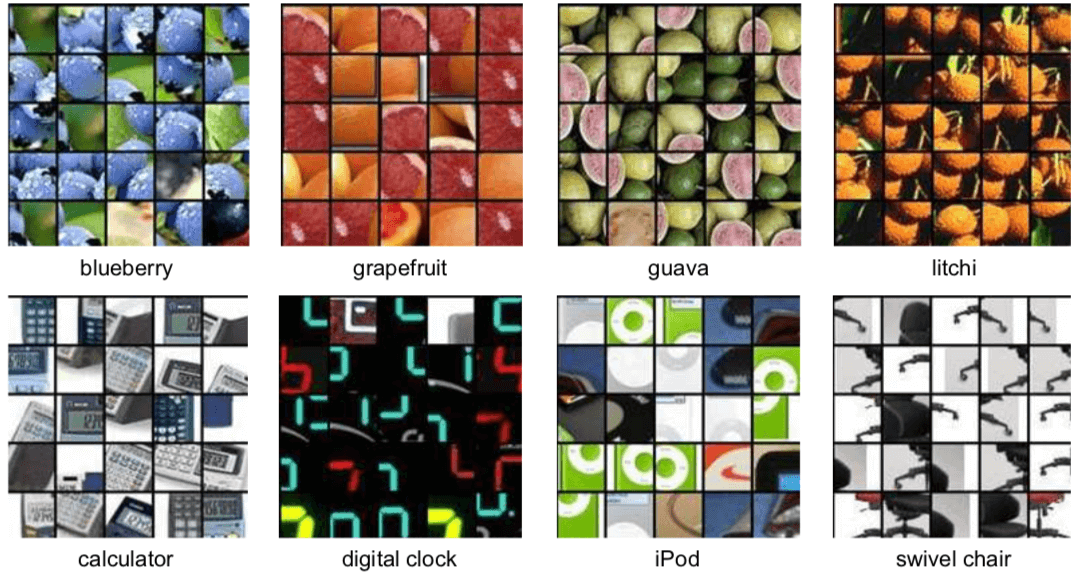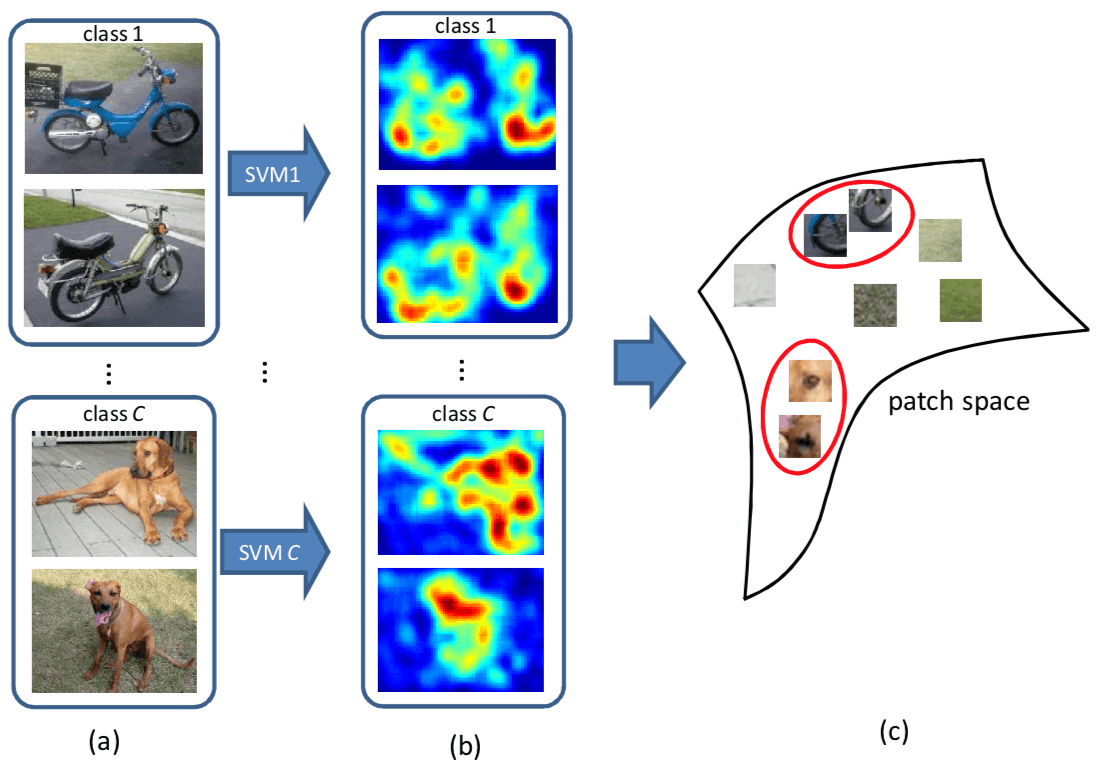Semantic Point Detector

ACM Multimedia 2011 的文章。

## MotivationWeakly Supervised Learning 的核心问题就在于怎么用 Weak label 上的 loss 来改善在具体任务上的 Prediction。这篇文章是怎么建立 Weak Label 和 Accurate Prediction 之间的关系的呢，具体地说，是怎么把 image-level classification 和 patch-level classification 给联系起来的呢？本文是用了两点，第一点是 image representation 是用所有 patch representation 的 linear combination，第二点是采用了 linear SVM 作为分类器。在给定 local patch descriptor $\phi \left( I _ { p } \right)$ 的情况下，image $I$ 的 Representation 就是

$$\Phi ( I ) = \frac { 1 } { P } \sum { p = 1 } ^ { P } \phi \left( I { p } \right)$$

$$f ( I ) = w ^ { T } \Phi ( I ) = \frac { 1 } { P } \sum { p = 1 } ^ { P } w ^ { T } \phi \left( I { p } \right) = \frac { 1 } { P } \sum { p = 1 } ^ { P } f \left( I { p } \right)$$

## Model

emmm… 好像 Model 上面说的差不多了，补充些细节。patch representation 用的是 Super-Vector coding，感兴趣的可以看原文的公式(1), (2), (3)。## Loss

Loss 就是正常的 SVM 的 hinge loss 啦，一个典型的正负类分类的 loss。